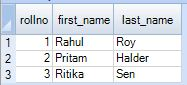Teradata Volatile tables act like a normal Teradata table but are volatile in nature. What does that mean?

The Volatile tables in Teradata are created by the user and used only for the duration they are logged into the Teradata system and dropped automatically by Teradata Manager once the user disconnects from the session. Dropping here means, dropping both the definition and data inserted into the volatile table.

### What is the use of Volatile Table?

Suppose you are working in the Teradata database for some performance analysis and you have to create quite a few tables for the same. One option is here to create normal Teradata tables, use those and drop them at the end. Another smart way for doing this is creating the volatile tables, using them, and logoff. Teradata automatically drops those tables once you disconnect from the session.

### Teradata Create Volatile Table Syntax

Following is the syntax for creating Teradata Volatile table.

```CREATE [SET | MULTISET] Volatile Table table_name
(
column1 datatype,
column2 datatype,
.
.
columnN datatype)
<index_definition>
ON COMMIT [Delete|Preserve] Rows;```

### Teradata Create Volatile Table Example

The following example will create a volatile table names student.

```CREATE VOLATILE TABLE student
(
rollno INT,
first_name varchar(20),
last_name varchar(20)
)
primary index (rollno)
ON COMMIT PRESERVE ROWS;```

You can see that in the above example, we have mentioned `ON COMMIT PRESERVE ROWS`. This will preserve the data once you insert the rows into it. The default value is `ON COMMIT DELETE ROWS`.

### Insert Data into Volatile Table

Let’s insert some records into the volatile table.

```INSERT INTO student values (1,'Rahul','Roy');
INSERT INTO student values (2,'Pritam','Halder');
INSERT INTO student values (3,'Ritika','Sen');```

### Select data from Volatile Table

Next, we will run the Select Statement into the Volatile table.

`Select * from Student order by rollno;`

Output:At last, if we disconnect from the current session and after re-logging, run the same select statement again, we will find that the table student does not exist anymore.

Output:

```Select * from Student order by rollno;
*** Failure 3807 Object 'Student' does not exist.
Statement# 1, Info =0
*** Total elapsed time was 1 second.```